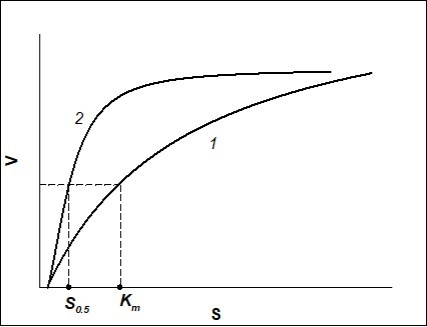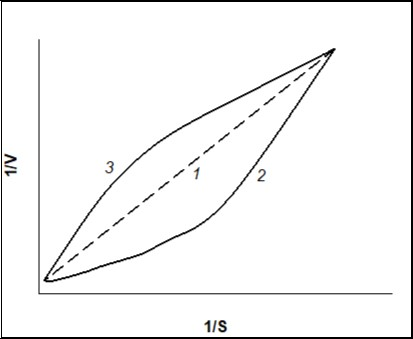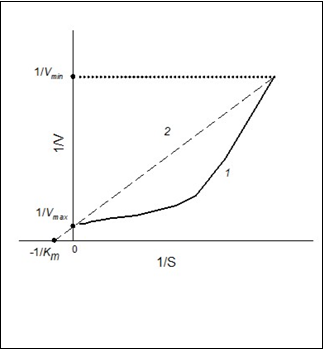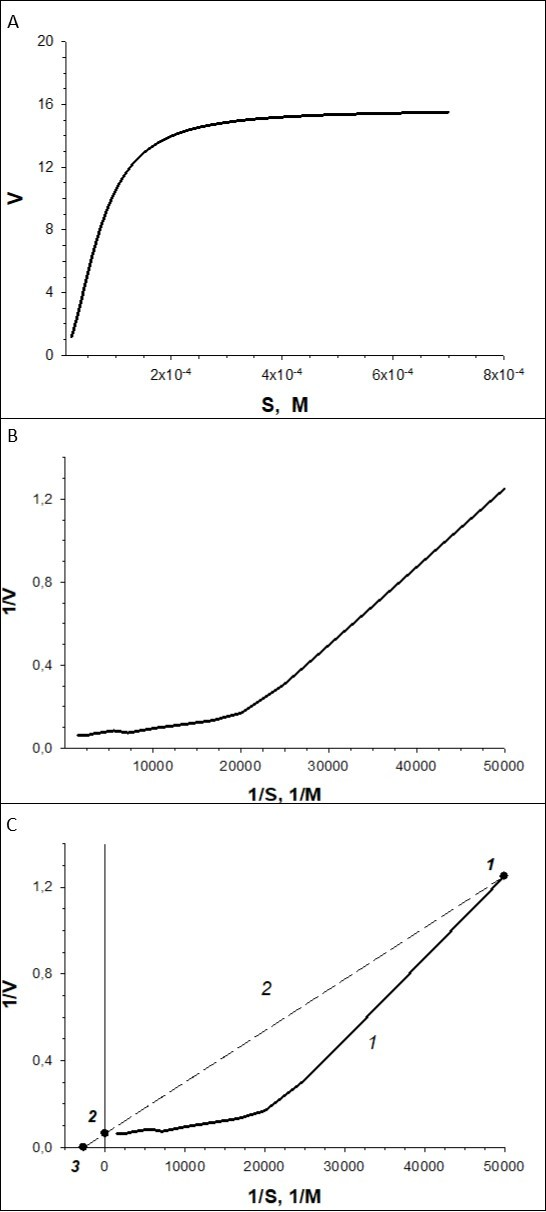### Journal of Biotechnology and Biomedical Science

Current Issue Volume No: 1 Issue No: 3

ISSN: 2576-6694

In Brief Open Access
• Available online freely Peer Reviewed
• ### Graphical Approach to Compare Concentration Constants of Hill and Michaelis-Menten Equations

Elena V. Emelyanova 1

1 FSBIS G.K. Skryabin Institute of Biochemistry and Physiology of Microorganisms, Russian Academy of Science, Pushchino, Moscow Region, Russia.

## Abstract

The aim of present study was to describe the graphical technique how to go from Hill concentration constant to Michaelis constant.

To compare enzymatic processes, the kinetics of which is subjected to different regularities, it is possible to use constants that characterize catalytic activity (Vmax) and concentration constants that are the substrate concentration at which the rate of the enzymatic process is equal to a half of maximum permissible rate. Concentration constants are S0.5 for Hill equation and Km for Michaelis-Menton equation. The graphical approach was proposed in order to go from concentration constant of Hill equation to Michaelis concentration of the process that could be characterized by the same catalytic activity (the same values of minimum and maximum rates) similar to that observed in the process described by Hill equation.

##### Author Contributions
Received 02 Aug 2018; Accepted 19 Sep 2018; Published 25 Sep 2018;

Checked for plagiarism: Yes

Review by: Single-blindThis is an open-access article distributed under the terms of the Creative Commons Attribution License, which permits unrestricted use, distribution, and reproduction in any medium, provided the original author and source are credited.

Competing interests

The authors have declared that no competing interests exist.

Citation:

Elena V. Emelyanova (2018) Graphical Approach to Compare Concentration Constants of Hill and Michaelis-Menten Equations. Journal of Biotechnology and Biomedical Science - 1(3):94-99.

## Introduction

There exist enormous amounts of research findings on the activity of various enzymes. As a result, these days a greater attention is focused on determination of the mechanisms of enzymatic reactions and kinetic constants. To find the constants for monomeric enzymes for which the dependence of the rate of a reaction, catalyzed by these enzymes, on substrate concentration is subjected to the hyperbolic kinetics 1, 2, 3, the Michaelis-Menten equation has to be used:

V = Vmax·S/(Km+ S),

Where

Vis the rate of a reaction catalyzed by the enzyme;

Sis a substrate concentration;

Vmax is maximum rate of enzymatic reaction (i.e. maximum value of the rate when S→∞);

Km is the Michaelis constant (Michaelis concentration), which is determined graphically as substrate (S) concentration at which V = 0.5Vmax and used for characterization of the affinity of the enzyme to a substrate.

The enzymes, the molecules of which consist of several identical monomers (subunits), are allosteric enzymes. A sigmoid (S-shape) but not Michaelis-Menten hyperbolic plot of the dependence of the rate of enzymatic reaction on the initial substrate concentration is typical of allosteric enzymes 4. A sigmoid dependence of the rate of enzymatic reaction on substrate concentration for allosteric enzymes can be described using empirical formula suggested by Hill 5:

V = Vmax·Sn/(S0.5n +Sn),

where

S0.5 is substrate (S) concentration at which V=0.5Vmax (Hill concentration constant);

n is Hill coefficient used for assessment of S-shape functions.

As to stable soluble enzymes of simple structure, the enzymatic activity can be determined in cell-free extracts of microorganisms. But some enzyme complexes can not remain active during cell destruction. So, the activity of these enzymes of multi-subunit structure cannot be determined in cell-free extract. The activity of these enzymes is assessed indirectly by the response of intact cells to enzyme substrate. This refers, for instance, to benzoate 1,2-dioxygenase. The activity of this enzyme is estimated by change in oxygen consumption by whole cells after injection of enzyme substrate (polarographic determination) 6, 7. A biosensor method can be also used in comparative assessment of dioxygenase activity. It is known, that oxygenase component of dioxygenase system from Pseudomonas putida mt-2 has an (alpha beta)(3) subunit structure 8. Notwithstanding multi-subunit structure of dioxygenase, the dependence of V vs. S could be of hyperbolic and/or S-shape form both at polarographic and biosensor determination 9.

How to compare the enzymes the kinetics of which is different? In a series of papers for the comparison of enzyme activity, the investigators went from Hill constant to Michaelis constant using the calculation formula. Hence, the use of the constants of hyperbolic dependency is necessary to calculate the inhibition constants 10.

Reasonable comparison facilitates understanding the mechanism of the process, but the unmotivated comparison has no results. For the comparison of two processes with different mechanisms the catalytic activities (Vmax) have to be compared. Concentration constants can also be compared: they are Michaelis constant (Michaelis concentration), which is determined graphically as substrate concentration at which the rate of enzyme reaction is half of Vmax, and substrate concentration S0.5 of Hill equation at which V=0.5Vmax.

Even having not estimated calculation formulas described in literature to go from S0.5 to Km it should be noted that complex mathematical manipulations are difficult for understanding. So, in this paper, a simple in use approach is suggested to go from Hill constant to Michaelis constant.

The coefficient n (Hill coefficient) is present in the Hill equation. The Hill coefficient is dimensionless. It should be taken into account, that the Hill coefficient is a parameter of an empirical formula and has no physical sense. Hill coefficient is not larger (≤) than the amount of active sites, although in terms of rather strong enzyme-substrate interactions this coefficient can be close proximity to the amount of active sites 4. In 9 it was shown that depending on the conditions of cultivation of the cells containing benzoate dioxygenase (allosteric enzyme), the Hill coefficient varied from 1 to 3. This coefficient could not be used in order to go from constant S0.5 of Hill equation to Michaelis constant, Km.

The Hill coefficient value (<1 or >1) characterizes a type of deviation from the Michaelis-Menten hyperbolic dependence: “negative” or “positive kinetic cooperativity” by substrate for allosteric enzyme. This coefficient is > 1 under “positive kinetic cooperativity” by a substrate and <1 under “negative kinetic cooperativity” (n = 1 for “classical” Michaelis-Menten hyperbolic dependency).

Negative kinetic cooperativity was observed, for example, for heterogeneous enzymatic preparations. Examples of this type of deviation from hyperbolic dependency are shown in 4. The author has no practical expertise in conducting a research under mentioned conditions, although there are prerequisites for implementation of negative cooperativity in the experiment.

The physiological significance of positive kinetic cooperativity lies in the fact that it is sufficient to have traces of a substrate to activate metabolism. Moreover, saturation is achieved at lower substrate concentrations; this is important for bacteria, for which toxic substances often serve as substrates 4. In this case, positive kinetic cooperativity by a substrate plays a protective role.

Under positive cooperativity, the catalytic efficiency of active sites (and the affinity to a substrate) of the allosteric enzyme increases as active sites are filled by a substrate. The greater is the affinity to a substrate; the lower is the value of a constant that characterizes the affinity to a substrate.

If V vs. S dependence of the process is depicted by S-shape curve, it is necessary to decide what parameters of the process (Michaelis-Menten kinetics), for which Km has to be calculated, should be. If for the process of Michaelis-Menten kinetics the maximum rate of enzyme-substrate reaction and minimum substrate concentration, to which the enzyme is sensible, should be similar to those of S-shape curve (positive kinetic cooperativity), then curves of V vs. S dependence for Michaelis-Menten kinetics and positive kinetic cooperativity (Hill equation) will be the same as shown in Figure 1.

Figure 1. Dependence of the rate of enzymatic reaction (V)on the initial substrate concentration (S) when Hill coefficient n=1 (Michaelis-Menten kinetics) (curve 1) and when n>1 (sigmoid curve described by Hill equation, positive kinetic cooperativity) (curve 2).Non-linearity of the dependency of 1/V on 1/S confirms that the V vs. S dependency is not hyperbolic. But if the 1/V on 1/S dependency is linear, V vs. S dependency is hyperbolic (Figure 2, line curve 1). Concavity of a curve of 1/V-1/S (Figure 2, curve 2)points to “positive kinetic cooperativity” by a substrate for the allosteric enzyme, but under “negative kinetic cooperativity” a curve of 1/V-1/S (Figure 2, curve 3) will be convex 11. Figure 3 represents thedependences of the rate of enzymatic reaction on the initial substrate concentration in reciprocal coordinates for Hill (positive kinetic cooperativity) and Michaelis-Menten equations.

Figure 2. Dependence of the rate of enzymatic reaction (V)on the initial substrate concentration (S) in reciprocal coordinates for “classical” Michaelis-Menten kinetics, n=1 (line curve 1), positive kinetic cooperative by a substrate, n>1 (curve 2), and negative kinetic cooperativity by a substrate, n<1 (curve 3).To go from S0.5 to Km, it is enough to plot a line similar to line 2 in Figure 3 using Sigma Plot program. The line has to pass through a point of S-shape curve which has maximum value of 1/V. This is a reciprocal value of the minimum rate (1/Vmin) of enzyme-substrate reaction, which can be detected.

Figure 3. Dependence of the rate of enzymatic reaction (V)on the initial substrate concentration (S) in reciprocal coordinates for positive kinetic cooperativity (1) and hyperbolic dependency (2).As a matter of fact, the line should pass through a cluster of points which are reciprocal to Vmax: this is a saturation area of the curve V vs. S. The line has to cross the axis 1/V at the point 1/Vmax, where Vmax is a known value. Vmax is the constant of the sigmoidal dependency (Hill equation). The equation of the plotted line is 1/V=a + b1/S, where a and b are positive values. In this case, 1/a = Vmax, and the required Michaelis concentration will be obtained when 1/V=0: Km = b/a. So, the estimated value of the Michaelis constant will be calculated for the process with the catalytic activity which is the same for the process with Hill kinetics. The example how to go from S0.5 to Km is given below.

## Example of Transformation (Figure 4).

With the use of experimental data we plot a curve of V vs. S (Figure 4A) dependence, which can be described by Hill equation V = Vmax·Sn/(S0.5n +Sn), where S0.5 = 69.789x10-6 mM = 69.789 µM, Vmax = 15.653 pA/s, n = 2.00. Then, the curve in reciprocal coordinates, 1/V-1/S, is plotted (Figure 4B). This curve will be concave. At the third step, we draw a line (Figure 4C) using the program. The line passes through the point 1, the coordinates of which are the maximum values of 1/V-1/S, and through point 2, coordinates of which are 1/S = 0 and 1/V = 1/Vmax = 1/15.653 = 0.06389. The program calculates the equation of the line curve plotted 1/V = a+bx1/S = 0.0639 + 2.3722x10-5 x1/S. The line crosses 1/S axis at point 3, the coordinates of which are 1/V = 0 and 1/S = -1/Km. Hence, Km = 2.3722/0.0639x10-5 = 371.2 µM.

Figure 4. Example of transformation.## Conclusion

With the graphical approach it is rather easy to go from concentration constant of Hill equation to Michaelis concentration.

## Cited by (1)

1.Ford Rochelle, Devereux Stephen. J., Quinn Susan. J., O'Neill Robert. D., 2019, Carbon nanohorn modified platinum electrodes for improved immobilisation of enzyme in the design of glutamate biosensors, The Analyst, 144(17), 5299, 10.1039/C9AN01085H